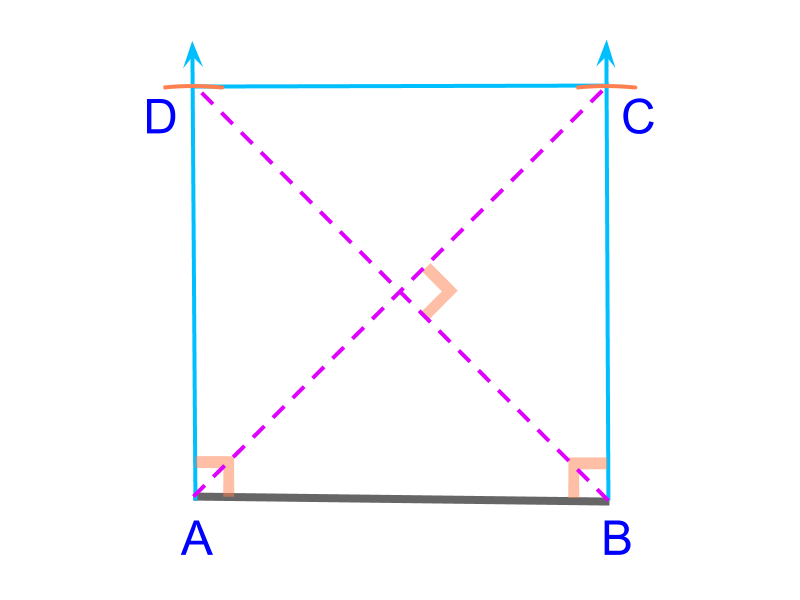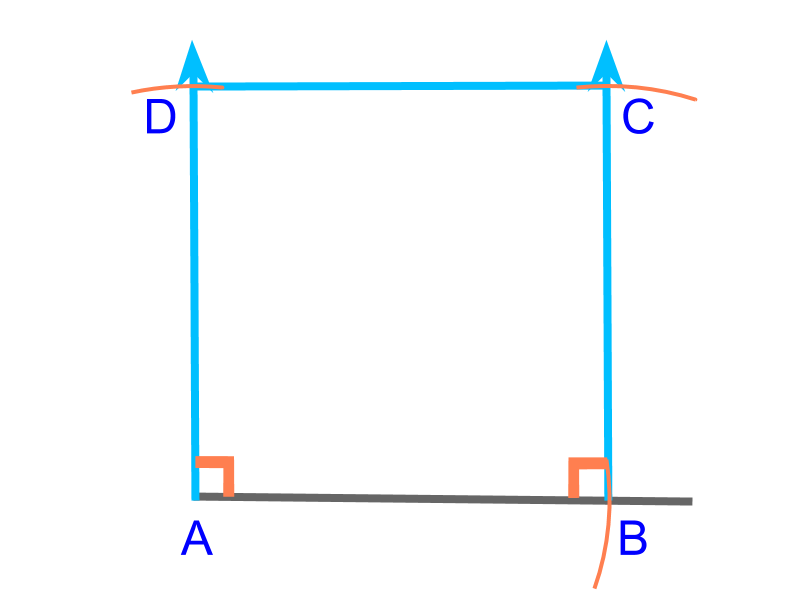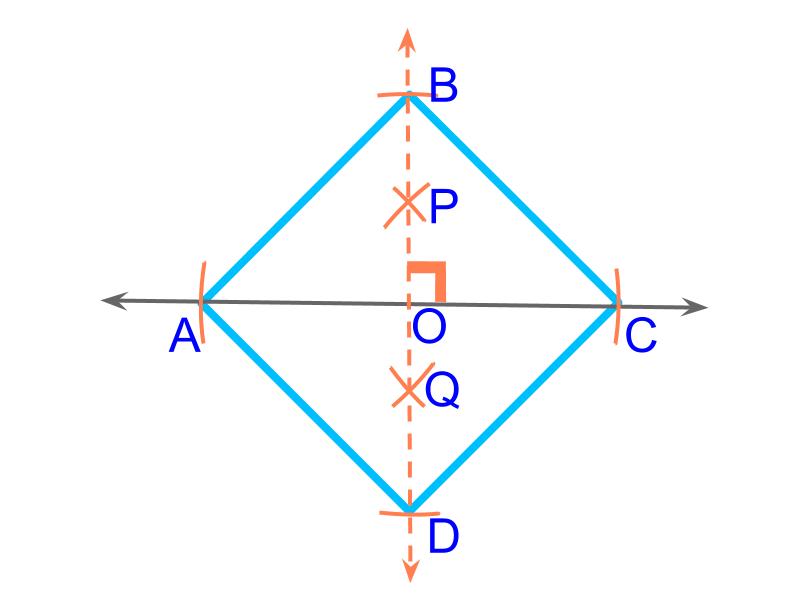maths > construction-basics

Construction of Square

what you'll learn...

overview

In this page, constructing squares is explained. It is outlined as follows.

•  Properties of squares is explained

•  The number of independent parameters in a square is $1$$1$

•  For a given parameter, construction of squares is approached as combination of triangles (sss, sas, asa, rhs, sal) and using the properties of squares.

understanding squareA square is a rectangle with all sides equal.

A rectangle is defined by $2$$2$ parameters. And in a square, the following properties provide additional dependency of parameters.

•  all sides are equal

•  diagonals are equal and perpendicularly bisect

•  opposite sides are parallel

•  all interior angles are ${90}^{\circ }$${90}^{\circ}$

•  two angles on diagonals are supplementary.

These properties cause one parameter to be dependent on other parameters and so, a square is defined by $1$$1$ parameter.To construct a square, the side ($\overline{AB}$$\overline{A B}$) is given. This is illustrated in the figure. To construct, consider this as two SAS triangles $ABC$$A B C$ and $ABD$$A B D$.To construct a square, the diagonal ($\overline{AC}$$\overline{A C}$) is given. This is illustrated in the figure.

To construct the specified square, "Consider as two SAS triangles and Mark the vertices at half diagonals on perpendicular lines". Use the property that diagonals bisect.

summaryConstruction of Square :

Properties of Square:

•  all sides are equal

•  diagonals are equal and perpendicularly bisect

•  opposite sides are parallel

•  all interior angles are ${90}^{\circ }$${90}^{\circ}$.

The formulations of questions

•  $1$$1$ side

•  $1$$1$ diagonal

use properties to figure out dependent parameters and look for triangles

Outline